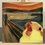# Heart power and ischemic heart disease

Ischemic heart disease is caused basically by an imbalance between $\mathrm{O}_2$ (which is translated into energy) demand and consumption by the heart. Therefore let us investigate how much energy the heart spends per unit of time and what factors contribute to such a demand.

As the blood flows out of the heart it is impelled against a pressure $p$ (the afterload). If the area of the exiting artery (the aorta) is $A$, then the force the heart must be exerting is

$F = p \cdot A.$

If the mean velocity the blood exits the heart is $v$ and the heart takes a time $t$ to do it, then we can multiply both sides of the last equation by $vt$ resulting in

$Fvt = p \cdot (Avt).$

Observe that $vt$ is also the distance travelled by the blood expelled by the heart in one pump. Therefore $Avt = V$ (in the right side of the equation) is the stroke volume (volume of blood expelled per beat) and $Fv = \Omega$ is the mean heart power. Therefore

$\Omega \cdot t = p \cdot V.$

The last thing to notice is that $t$ is the time of a full-cycle. Therefore if $f$ is the cardiac frequency, then $t = 1/f$ and we can finally write

$\Omega = pVf.$

This means that the energy used by the heart increases linearly with the afterload, the stroke volume and the heart frequency. This explains why unstable anginas happen when we exercise, for exemple.

Some medications such as nitrates decrease the heart power by decreasing the heart's stroke volume by accumulating blood in the veins (therefore less blood reaches the heart). Other medications such as beta-blockers decrease the heart power by blocking beta-1 receptors and therefore decreasing the heart frequency.

The most important thing to notice is that the percentage of decrease in heart power is exactly the same as the percentage decrease in the mechanism the medication is working on.Note by Lucas Tell Marchi
3 weeks, 1 day ago

This discussion board is a place to discuss our Daily Challenges and the math and science related to those challenges. Explanations are more than just a solution — they should explain the steps and thinking strategies that you used to obtain the solution. Comments should further the discussion of math and science.

When posting on Brilliant:

• Use the emojis to react to an explanation, whether you're congratulating a job well done , or just really confused .
• Ask specific questions about the challenge or the steps in somebody's explanation. Well-posed questions can add a lot to the discussion, but posting "I don't understand!" doesn't help anyone.
• Try to contribute something new to the discussion, whether it is an extension, generalization or other idea related to the challenge.

MarkdownAppears as
*italics* or _italics_ italics
**bold** or __bold__ bold
- bulleted- list
• bulleted
• list
1. numbered2. list
1. numbered
2. list
Note: you must add a full line of space before and after lists for them to show up correctly
paragraph 1paragraph 2

paragraph 1

paragraph 2

[example link](https://brilliant.org)example link
> This is a quote
This is a quote
    # I indented these lines
# 4 spaces, and now they show
# up as a code block.

print "hello world"
# I indented these lines
# 4 spaces, and now they show
# up as a code block.

print "hello world"
MathAppears as
Remember to wrap math in $$ ... $$ or $ ... $ to ensure proper formatting.
2 \times 3 $2 \times 3$
2^{34} $2^{34}$
a_{i-1} $a_{i-1}$
\frac{2}{3} $\frac{2}{3}$
\sqrt{2} $\sqrt{2}$
\sum_{i=1}^3 $\sum_{i=1}^3$
\sin \theta $\sin \theta$
\boxed{123} $\boxed{123}$